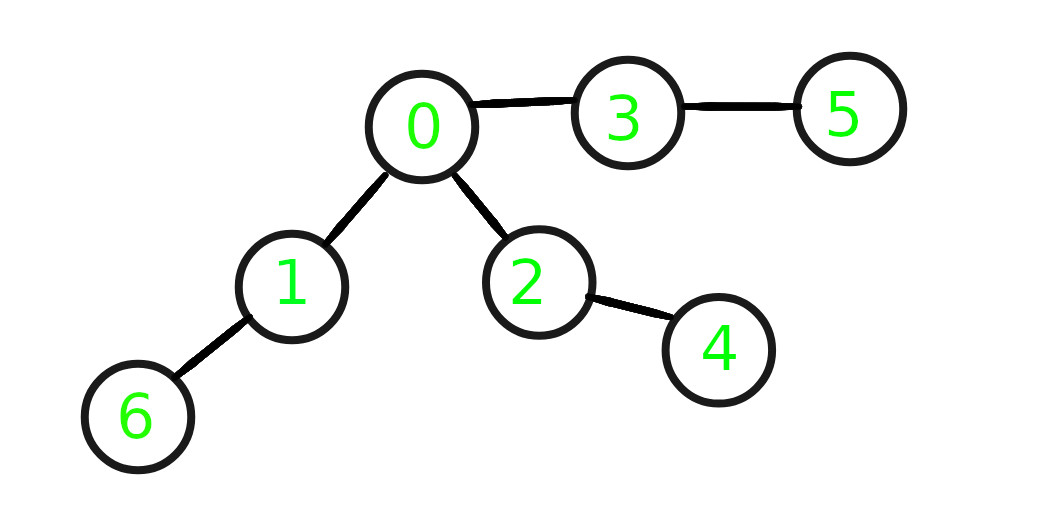# Sum of minimum element at each depth of a given non cyclic graph

Given a non-cyclic graph having V nodes and E edges and a source node S, the task is to calculate the sum of the minimum element at each level from source node S in the given graph.

Examples:

Input: S = 0, Below is the given graphOutput: 5
Explanation:
There is only one node at depth 0 i.e. 0.
At depth 1 there are 3 nodes 1, 2, 3, and minimum of them is 1.
At depth 2 there are another 3 nodes i.e. 6, 4, 5, and a minimum of them is 4.
So the sum of minimum element at each depth is 0 + 1 + 4 = 5.

Input: S = 2, Below is the given graphOutput: 8
Explanation:
At depth 0 only 1 node exists i.e. 2.
At depth 1 minimum element is 0.
At depth 2 minimum element is 1.
At depth 3 minimum element is 5
So the sum of minimum element at each depth is 2 + 0 + 1 + 5 = 8.

## Recommended: Please try your approach on {IDE} first, before moving on to the solution.

Approach: The idea is to use DFS Traversal. Below are the steps:

1. Initialise an array(say arr[]) to store the minimum element at each level.
2. Start the DFS Traversal from the given source node S with a variable depth(initially 0).
3. Update the minimum value of current depth in the array arr[].
4. Recursively recurr for child node with incrementing the value of depth from the previous recursive call such that the minimum value at corresponding depth can be updated accordingly.
5. After the above steps the sum of values stored in arr[] is the required total sum.

Below is the implementation of the above approach:

## C++

 `// C++ program for the above approach ` `#include ` `using` `namespace` `std; ` ` `  `// Function to add an edge in a graph ` `void` `addEdge(vector<``int``> adj[], ` `            ``int` `u, ``int` `v) ` `{ ` `    ``adj[u].push_back(v); ` `    ``adj[v].push_back(u); ` `} ` ` `  `// Variable to store depth of graph ` `int` `max_depth = 0; ` ` `  `// Function to know the depth of graph ` `void` `find_depth(vector<``int``> adj[], ` `                ``vector<``bool``>& visited, ` `                ``int` `start, ``int` `depth) ` `{ ` `    ``// Mark the node start as true ` `    ``visited[start] = ``true``; ` ` `  `    ``// Update the maximum depth ` `    ``max_depth = max(max_depth, depth); ` ` `  `    ``// Recurr for the child node of ` `    ``// start node ` `    ``for` `(``auto` `i : adj[start]) { ` `        ``if` `(!visited[i]) ` `            ``find_depth(adj, visited, ` `                    ``i, depth + 1); ` `    ``} ` `} ` ` `  `// Function to calculate min value ` `// at every depth ` `void` `dfs(vector<``int``> adj[], ``int` `start, ` `        ``vector<``bool``>& visited, ` `        ``vector<``int``>& store_min_elements, ` `        ``int` `depth) ` `{ ` `    ``// marking already visited ` `    ``// vertices as true ` `    ``visited[start] = ``true``; ` ` `  `    ``// Store the min value for ` `    ``// every depth ` `    ``store_min_elements[depth] ` `        ``= min(store_min_elements[depth], ` `            ``start); ` ` `  `    ``// Traverse Child node of start node ` `    ``for` `(``auto` `i : adj[start]) { ` `        ``if` `(!visited[i]) ` `            ``dfs(adj, i, visited, ` `                ``store_min_elements, ` `                ``depth + 1); ` `    ``} ` `} ` ` `  `// Function to calculate the sum ` `void` `minSum_depth(vector<``int``> adj[], ` `                ``int` `start, ` `                ``int` `total_nodes) ` `{ ` `    ``vector<``bool``> visited(total_nodes, ` `                        ``false``); ` ` `  `    ``// Calling function to know ` `    ``// the depth of graph ` `    ``find_depth(adj, visited, ` `            ``start, 0); ` ` `  `    ``// Set all value of visited ` `    ``// to false again ` `    ``fill(visited.begin(), ` `        ``visited.end(), ``false``); ` ` `  `    ``// Declaring vector of ` `    ``// "max_depth + 1" size to ` `    ``// store min values at every ` `    ``// depth initialise vector ` `    ``// with max number ` `    ``vector<``int``> store_min_elements( ` `        ``max_depth + 1, INT_MAX); ` ` `  `    ``// Calling dfs function for ` `    ``// calculation of min element ` `    ``// at every depth ` `    ``dfs(adj, start, visited, ` `        ``store_min_elements, 0); ` ` `  `    ``// Variable to store sum of ` `    ``// all min elements ` `    ``int` `min_sum = 0; ` ` `  `    ``// Calculation of minimum sum ` `    ``for` `(``int` `i = 0; ` `        ``i < store_min_elements.size(); ` `        ``i++) { ` `        ``min_sum += store_min_elements[i]; ` `    ``} ` ` `  `    ``// Print the minimum sum ` `    ``cout << min_sum << endl; ` `} ` ` `  `// Driver Code ` `int` `main() ` `{ ` `    ``// Given Nodes and start node ` `    ``int` `V = 7, start = 0; ` ` `  `    ``// Given graph ` `    ``vector<``int``> adj[V]; ` `    ``addEdge(adj, 0, 1); ` `    ``addEdge(adj, 0, 2); ` `    ``addEdge(adj, 0, 3); ` `    ``addEdge(adj, 1, 6); ` `    ``addEdge(adj, 2, 4); ` `    ``addEdge(adj, 3, 5); ` ` `  `    ``// Function Call ` `    ``minSum_depth(adj, start, V); ` `} `

Output:

```5
```

Time Complexity: O(V + E)
Auxillary Space: O(V)

Attention reader! Don’t stop learning now. Get hold of all the important DSA concepts with the DSA Self Paced Course at a student-friendly price and become industry ready.

My Personal Notes arrow_drop_upCheck out this Author's contributed articles.

If you like GeeksforGeeks and would like to contribute, you can also write an article using contribute.geeksforgeeks.org or mail your article to contribute@geeksforgeeks.org. See your article appearing on the GeeksforGeeks main page and help other Geeks.

Please Improve this article if you find anything incorrect by clicking on the "Improve Article" button below.

Improved By : ronak037